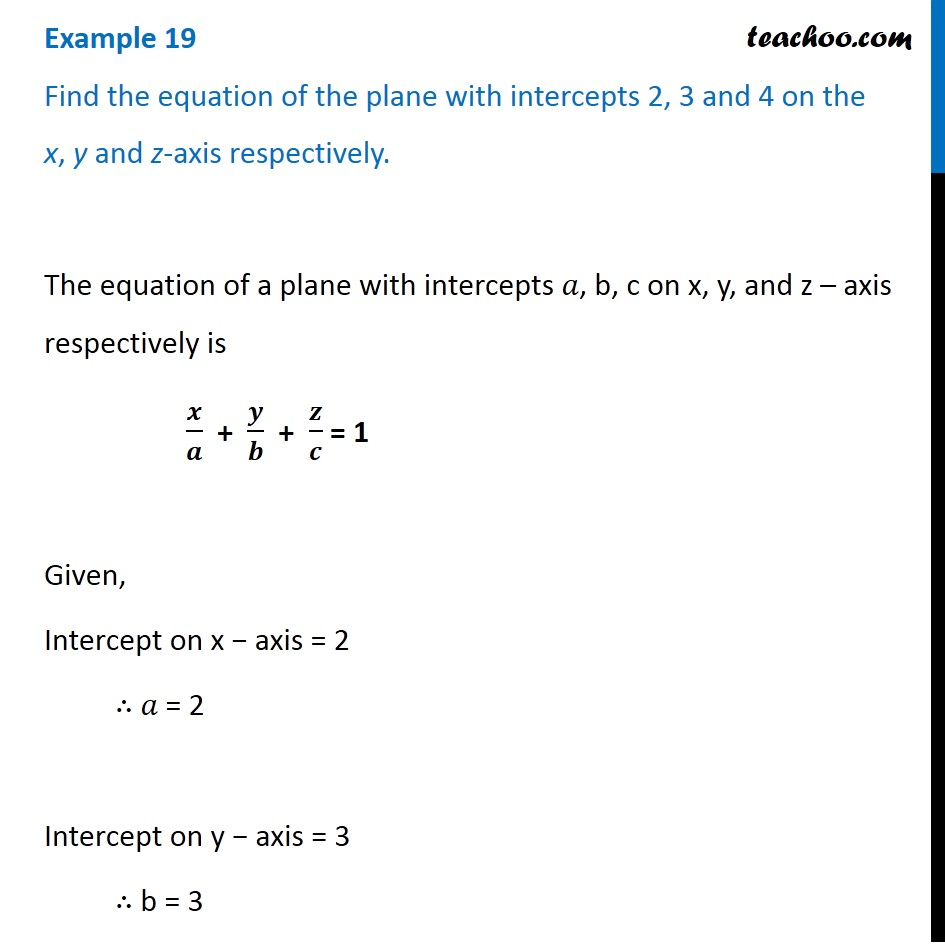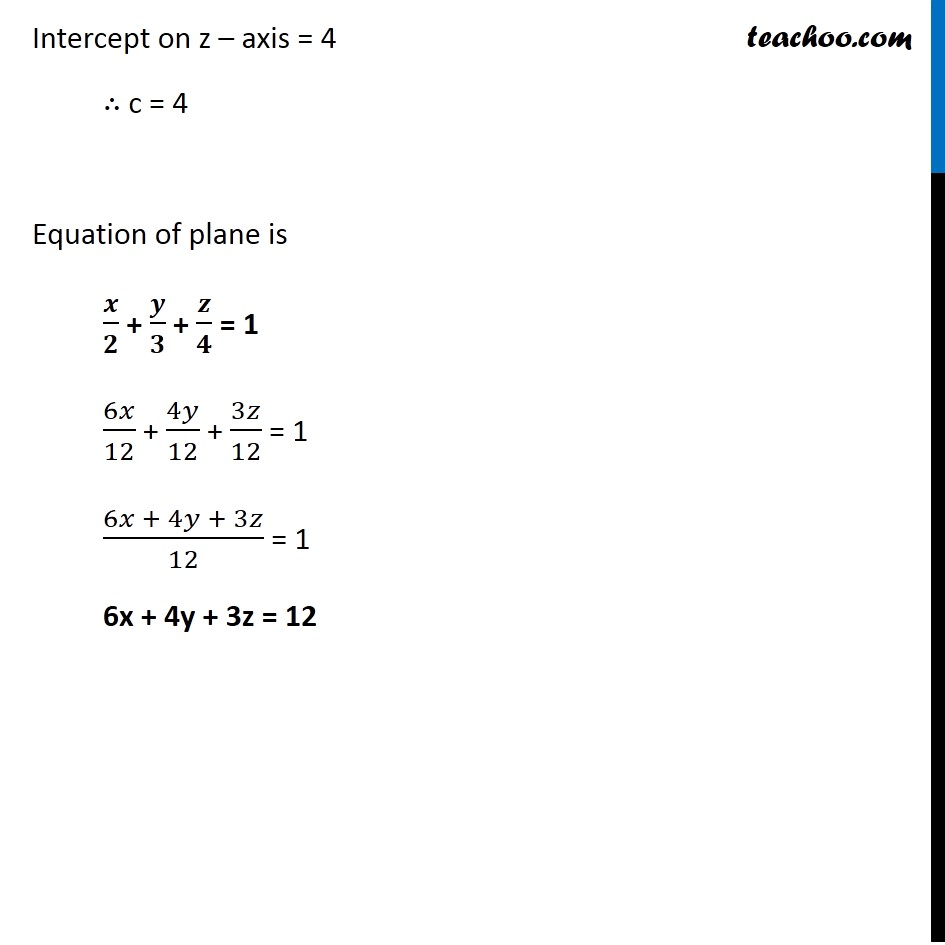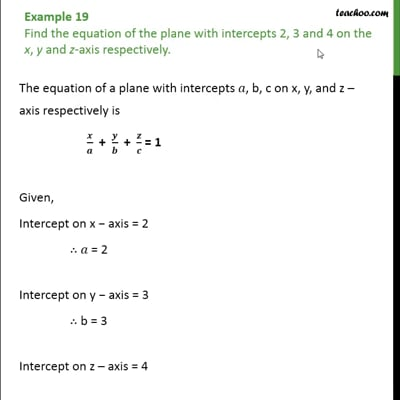Examples

Chapter 11 Class 12 Three Dimensional Geometry
Serial order wiseThis video is only available for Teachoo black users

Introducing your new favourite teacher - Teachoo Black, at only ₹83 per month

### Transcript

Example 19 Find the equation of the plane with intercepts 2, 3 and 4 on the x, y and z-axis respectively. The equation of a plane with intercepts 𝑎, b, c on x, y, and z – axis respectively is 𝒙/𝒂 + 𝒚/𝒃 + 𝒛/𝒄 = 1 Given, Intercept on x − axis = 2 ∴ 𝑎 = 2 Intercept on y − axis = 3 ∴ b = 3 Intercept on z – axis = 4 ∴ c = 4 Equation of plane is 𝒙/𝟐 + 𝒚/𝟑 + 𝒛/𝟒 = 1 6𝑥/12 + 4𝑦/12 + 3𝑧/12 = 1 (6𝑥 + 4𝑦 + 3𝑧)/12 = 1 6x + 4y + 3z = 12